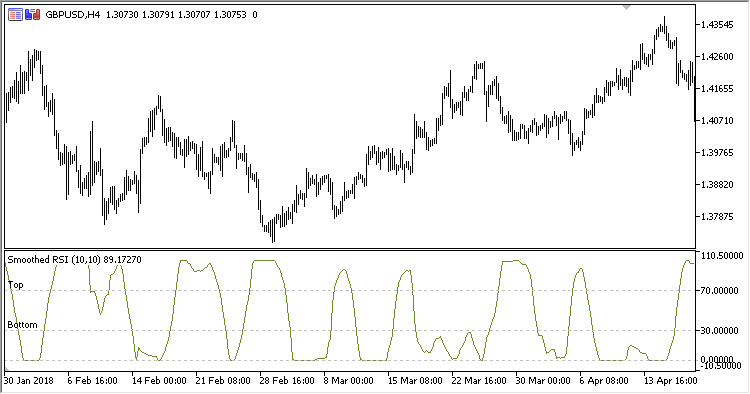Interesting script?
So post a link to it -
let others appraise it

You liked the script? Try it in the MetaTrader 5 terminal# Smoothed_RSI - indicator for MetaTrader 5

Views:
1031
Rating:
Published:
2018.12.18 19:15

Smoothed RSI indicator. The indicator is based on moving average data.

There are seven configurable parameters:

• Source period - source data period for calculation (МА)
• Source method - source data calculation method (МА)
• Source applied price - source data calculation price (МА)
• Smoothed RSI period - smoothing period
• Smoothed RSI method - smoothing method
• Top level - upper level
• Bottom level - lower level

Calculation:

SmRSI = 100.0-(100.0 / (1.0+AvgPositive / AvgNegative))

where:

AvgPositive = MA(Pos, Smoothed RSI period, Smoothed RSI method)
AvgNegative = MA(Neg, Smoothed RSI period, Smoothed RSI method)

• If Diff > 0
Pos = Diff, Neg = 0
• If Diff < 0
Pos = 0, Neg = -Diff

Diff = MA - Close
MA - MA(Source applied price, Source period, Source method)

Compared to standard RSI, this indicator has a feature: When the price falls, SmRSI rises and vice versa.Translated from Russian by MetaQuotes Software Corp.
Original code: https://www.mql5.com/ru/code/22510SRSI

Slow RSI indicatorTime_Averaged_Price

Time Averaged Price indicatorSineWMA

Sine Weighted MA indicatorNormalized_Moving_Average_Slope

Normalized Moving Average Slope indicator